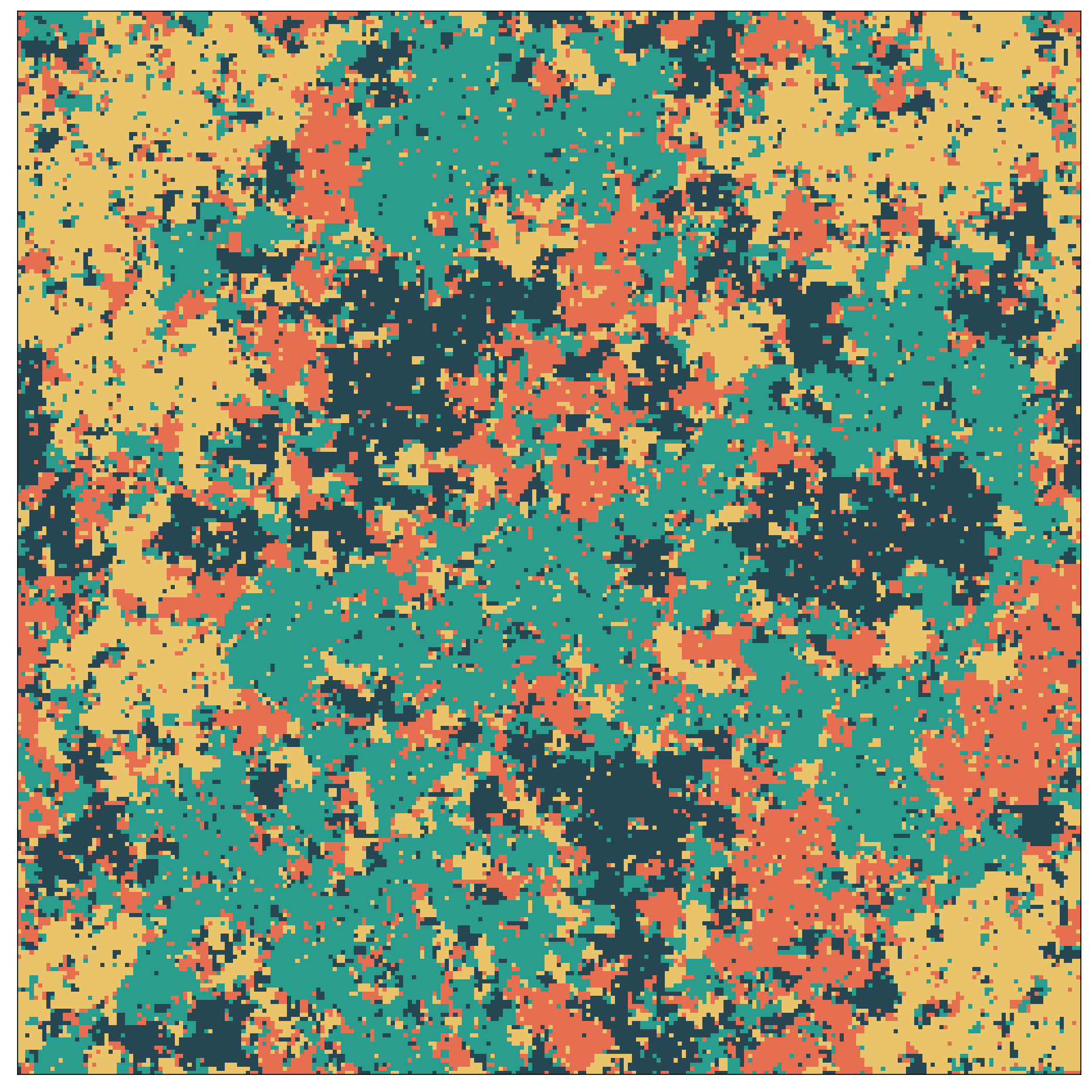Figure 1. Critical configuration of the 4-state Potts model.

The $$q$$-state Potts model1 represents a generalization of the Ising model, where spins can take on $$q$$ values, rather than only two. The Hamiltonian is given by

$\mathcal{H} = -J\sum\limits_{\langle i,j\rangle}\delta_{\phi_i,\phi_j},$

where $$J>0$$, $$\phi_i\in{0,1,\ldots,q-1}$$ and $$\delta$$ represents the Kronecker delta. In 2D, the ferromagnetic Potts model is known to exhibit a second-order phase transition for $$q \leq 4$$ and a first-order phase transition for $$q\geq 5$$.

What makes the Potts model particularly interesting is the fact that its critical properties can be calculated exactly2. The transition temperature is, for example given by the expression

$\beta_c(q) = \frac{1}{2}\log\left(1+\sqrt{q}\right)$

and the critical exponents are simple fractions, such as $$\nu = 2/3$$ and $$\beta = 1/12$$ for $$q=4$$. We verify these exponents by simulating the model using MARQOV, resulting in the following data collapse of the magnetization, which is given by

$\langle m \rangle = \frac{q\max{n_i}-N}{N(q-1)}$

where $$n_i \leq N$$ denotes the number of spin with configuration $$i = 1,\ldots, q$$. Even though we invested only comparably little CPU time in this example, the data collapse turns out very convincing.Figure 2. Scaling collapse of the magnetization for the 4-state Potts model.

1. Wu, F. Y. (1982). The Potts model. Reviews of modern physics, 54(1), 235.
2. Baxter, R. J. (1982). Exactly solved models in statistical mechanics. Academic Press Limited, London.